Data is in and data is hot, because data is valuable. The big data revolution has demonstrated that the modern, interconnected world is brimming with data-based insights waiting to be revealed. That’s where data analysis methods come into play.

Whether you’re self-employed, work at a small business, or partake in the corporate world, it’s likely you will see data analysis in action. And even if you’re not charged with analyzing the data yourself, it’s still a good idea to know the various methods, tools, and reasons behind data-based insights. (You might even want to become a data scientist, regardless of your background.)

At the end of this article, I mention some caveats that will impact everyone. Even those who are not performing the actual calculations would benefit from understanding these caveats, why they arise, and what they mean.

## Background and Preliminary Concerns

Let’s first take a look at some concepts you must consider before you start with any kind of statistical analysis, machine learning, or computerized analysis.

### Qualitative vs. Quantitative Analysis

Qualitative data analysis is much more subjective and attempts to discover reasons, motivations, and feelings of the subjects of observation. Qualitative data collection might manifest in the form of interviews or free-response questionnaires.

If you want to know why customers don’t like your product, why your employees are leaving en masse, or why voters choose the candidates they do, qualitative analysis will be integral to your study.

On the other hand, quantitative data analysis looks at any quantifiable object, behavior, or characteristic and uses statistical methods to gain insight. Big data is all about quantitative analysis, because computers talk in numbers, not human cognitions and experiences.

If your task involves any kind of quantifiable aspect, like temperature, time spent on a section of a website, geographical distributions, or amounts of money, you will benefit from quantitative analysis.

Policy and business decisions usually combine the two: perhaps by looking at the geographic distribution of voters and customers, cross-referencing that with quantitative economic and behavior data, then conducting interviews and open-ended questionnaires to gather human experience, thoughts, and needs.

### Types of Variables

There are several types of variables, but the three main types are continuous, categorical, and binary (a special type of categorical).

Continuous variables can take any numeric value. Checking account balances are a continuous variable, because there are no gaps between one possible balance and the next. So \$100.05 is one possibility, as is \$100.06 or \$100.07. Sometimes these are termed numeric data. Time is a very frequently used continuous variable, as are temperature, number of barrels of oil consumed, and the processing power of a data center.

Conversely, categorical variables cannot be graphed as a line, but are better graphed as bars. Checking account balances can also be represented categorically, where the categories are \$100-\$999, \$1,000-\$9,999, \$10,000+. It doesn’t matter whether the account in question has \$101, \$983.01, or \$532.53 in it, it will fall into the same category. This differs from the continuous interpretation of account balances, where \$532.53, \$983.01, and \$101 are separated from each other.

Categorical variables need not be numeric; colors, types of material, marital status, and professions are all categorical variables that are not numbers.

Binary variables are quite common, and they’re categorical variables that have only two options (categories). On/off, color/monochrome (for printing), or open/closed are common binary variables.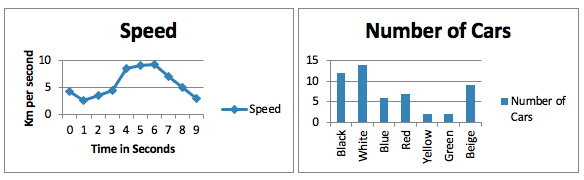The left graph shows two continuous variables (time and speed), while the right graphs a categorical variable (color) against a continuous variable (count of cars of a certain color)

### Data Cleaning

Before you can even begin your quantitative analysis, you need to clean the data. The “messiness” of your data will depend on how it was collected. Categorical data collected from multiple-choice customer surveys from a single source will be very clean.

On the other hand, manually entered data aggregated from 10 government agencies, all with different formats and functions, will require you to identify and remove errors, convert different scales, and format data to ensure consistency.

Entries might be missing or entered incorrectly. A sensor might be malfunctioning, or the receiving algorithm might be mangling the format. There might be duplicates or scaling factors that shouldn’t be there (such as accidentally multiplied by 10). If both data sets tabulate the number of employees, but one set is quoted in 000s while the other is quoted in raw numbers, the first data set will look 1,000 times smaller than it should when compared.

Data cleansing is itself a huge part of data analysis—cleaning and preparing data is up to 80% of the work of data science—and thus lies outside the scope of this article. It is also regarded as some of the most boring work, since you don’t get any insights from it. However, it is extremely important to have clean, prepared data. Otherwise the output is useless. (Check out this guide for a crash course introduction to data cleansing.)

## Statistical Indicators

Let’s get to the actual data analysis methods. There are many ways to analyze data. We’ll start with statistics, as these can give a lot of information about the data you already have. Then we’ll look at distributions and probabilities.

### Sample Size

Sometimes we can’t gather data on 100% of the population because the logistics are impossible or it’s simply too expensive. For those cases, we use a random sample, which is intended to randomly choose a representative subset from the whole set. This would be useful in political polls or customer surveys. You can’t force people to participate in surveys. Global atmospheric temperatures is another case. We simply cannot measure every single point on Earth, but we can take a representative sample.

At other times, we can look at the entire population. Sensor data from every sensor in a connected factory is one example. Population censuses are another.

Whether you have a sample or the entire population, let’s look at the various representative statistics, what they mean, and when to use them.

### Confidence Intervals and Margins of Error

When calculating a sample size, it’s important to note the target margin of error and the confidence interval. The margin of error is the precision, often quoted by news sources and spokespeople as “within plus/minus 3%” (when the margin of error is 3%).

The confidence interval shows how likely the sample truly reflects the population. For example, a confidence interval of 50% means the study will only capture the true outcome for the whole population half the time it is conducted. That’s pretty bad!

But a confidence interval of 99.8% is so close to 100% that you will be “sampling” nearly the whole population. If your sample size equals your population, you have a perfect chance of calculating an accurate measure, because you’ve asked everyone (or everything) in the population!

### Mean, Median, and Mode

These are the three most basic and common statistical indicators. Many people learn about them in high school math classes. They reflect a data set in a single number.

The mean (also termed average) is simply the center point of the data set. You add all the data points together and divide by the number of points. That’s it.

The median is another center point of the data set, but this is based on the counts, not the numbers themselves. You line up all the numeric data in ascending (or descending) order and find the point in the middle.

The mode is the most frequent data point. Modes are more applicable to categorical data, but they can be used for continuous data too. Every time the same number or category appears, its count is incremented by one. Once all the data points are analyzed, the one with the highest count is the mode. This is useful for finding common or popular categories (or numbers).

You may ask, “What is the difference between median and mean?” The mean can be skewed by outliers, and we can use the difference between the median and mean to spot potentially misleading issues.

Consider these two data sets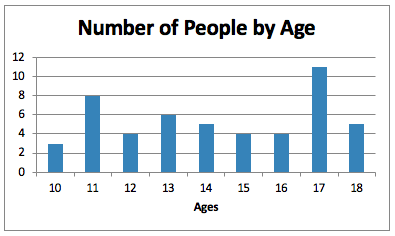Mean: 14.3, Median: 14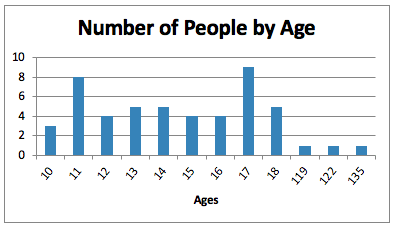Mean: 20.88, Median: 15.5

In the first data set, the median and mean are almost the same, indicating a pretty evenly spread distribution. But in the second set, the mean is much higher. It is actually greater than the ages of 47 of the 50 participants. That’s not very representative. For the second set, the median better encapsulates the overall distribution, while the mean is misleading.

If we look at a scatterplot of these two data sets (the participant number on the bottom, the age on the vertical axis), we can see how the second one has outliers and the consequent scaling crushes all the other data against the bottom: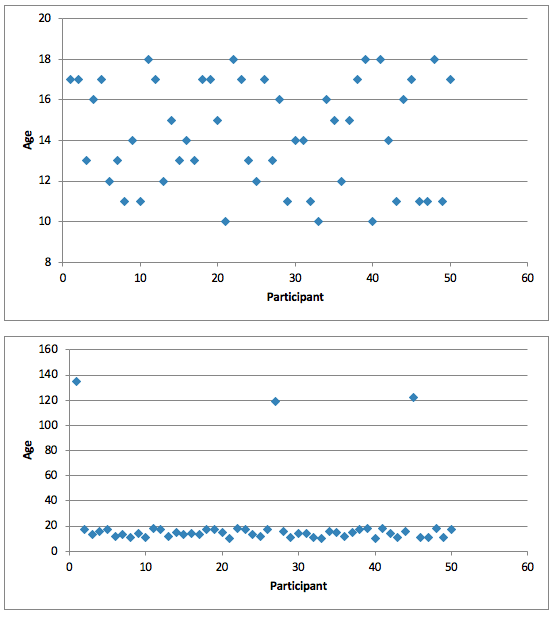Let’s also consider this data set: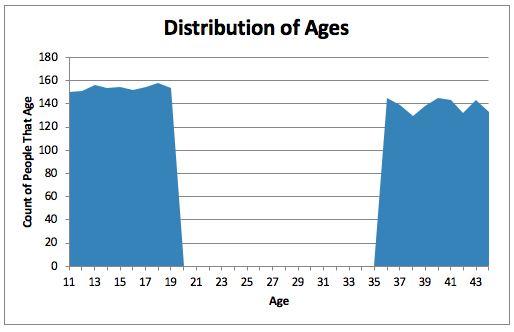Median: 19, Mean: 26.86

Here, we are misled by both measures. The median may lead careless observers to believe the population is younger, while no participants are at, or even within seven years of, the average! This is why it’s very important to know how your data is distributed and not rely solely on single-measure statistics.

### Types of Distributions

This is not a statistical indicator in itself, but it can heavily influence indicators, as we just witnessed. The most common types of distributions are uniform, normal, bimodal, and multimodal, but there are many, many more types.

Uniform distributions are (mostly) evenly spread out. Normal distributions, also called bell curves, have a peak in the center (centered by design at the mean). Bimodal distributions have two peaks. If you think about the mode in a bimodal distribution, the distribution has two main modes, or two very common outcomes.

### Standard Deviations

The standard deviation is a measure of how spread out the distribution is. The higher the standard deviation, the more spread out the data is. In normal distributions, roughly 68% of the data lies within one standard deviation of the mean.

You can use this measure to determine how unlikely a specific data point is. If the data point is within one standard deviation of the mean, it is a pretty likely data point. However, if the data point lies four standard deviations away from the mean, it’s probably an abnormality. It could just be an outlier or it could be a data error worthy of investigation.

### Ranges, Outliers, and -tiles

The range of the data is the difference between the highest and the lowest value in the set. Since this is using numbers, it is only applicable to numeric data (though not necessarily continuous—recall our categories of account balances above).

Sometimes there are outliers, which are many standard deviations away from the mean. These will skew the range and are often dropped during analysis. If all incomes in a geographic area are between \$40,000 and \$70,000 (the range), but one is \$750,000, the \$750,000 is an outlier. A representative range would exclude the outlier.

Percentiles are a good way to represent where in the distribution a data point lies. A data point at the 50th percentile is completely average, while one at the 98th percentile is extraordinary. Percentiles are cumulative, so a data point at the 98th percentile scores greater than 98% of all other data points in whatever variable is being investigated. A data point at the second percentile scores higher than only 2% of the data points.

We can also split up data sets into quartiles or quintiles to create categories for simpler explanations. If a data point appears in the fifth quintile, it falls above 80 on a range from 0 to 100. You can just as easily use other ranges, though. The first quartile of the range \$0-\$20,000 is \$0-\$4,999, so \$985 would fall into the first quartile.## Predictive Methods

If you want to predict the output value of some process or phenomenon, you can use predictive methods. The most common is regression analysis, which comes in a few flavors, of which we will explore four: linear, polynomial, multiple, and logistic. Regression looks at the relationship between the independent and dependent variables. The former is the input, or driver, of the process or function, while the latter is the output.

Regression will produce a line for visual interpretation, and it also produces a mathematical function that can take new inputs and predict the output.

### Linear Regression

Linear regression identifies a straight line to represent the relationship. It works well for data that has an inherent linear relationship, such as temperature and pressure. As temperature rises, so does pressure, and the relationship is pretty smooth. There aren’t peaks and valleys in the relationship between pressure and temperature.

The linear regression algorithm works by finding the line that is closest to the data points on average. For each data point, the algorithm finds the distance between the data point and a sample line. Then all the distances are added together and the algorithm generates a new sample line and remeasures all the distances. Whichever sample line has the lowest sum of distances is the chosen line.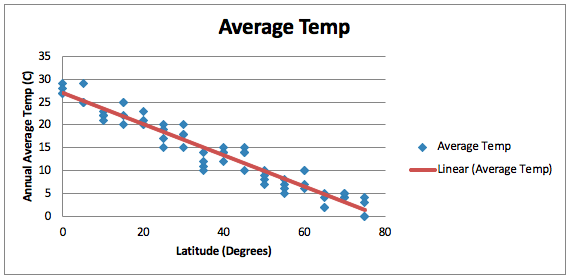Using the latitude of the location, we can predict somewhat accurately what the average temperature would be at that location. (The data here is completely fictitious). The red line is the output of the linear regression function. If we are at the 20 degree north latitude, we can expect the average annual temperature to be about 20 C.

### Polynomial Regression

Since linear regression assumes the relationship is linear, it does not work well on all data. Sometimes the relationship is positive (i.e., a rise in X is a rise in Y), while sometimes the relationship for the same set is negative (a rise in X correlates to a fall in Y). The positivity or negativity of the relationship fluctuates throughout the range.

These types of relationships are better represented by polynomial regression, which uses a polynomial (x2, x3, or xn functions). The algorithm follows the same method, but instead of fitting a line, it tries to use curves.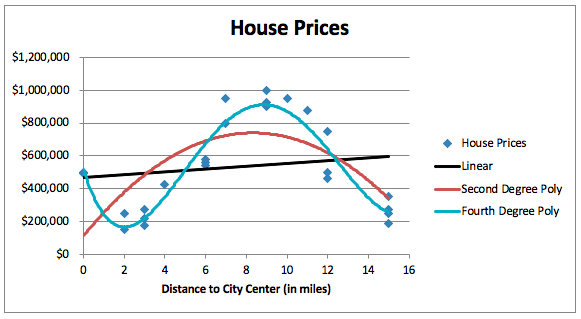The second degree polynomial regression line (x2 function) shows rising prices until about eight miles from the center, then the price falls again. The fourth degree polynomial regression (x4 function) shows a clear pattern of high prices in the very center, lower in an outer urban ring, a spike in the suburbs, and then a drop off as the area becomes rural. The linear trendline fails to capture either of these patterns.

### Underfitting and Overfitting

When using some techniques, like polynomial regression, it is possible to overfit the data. This means the predictive model (the polynomial line) too closely matches the sample data. This is a problem because it only matches the sample data, and when applied to similar but new data, it fails to accurately predict the outcome.

If we keep increasing the degree of the polynomial regression line, we will get more curves in the line and hence a tighter fit, but we should always be cautious to not overfit. Above, the fourth degree is probably acceptable if a sanity check indicates the predicted pattern matches reality.

Conversely, underfitting can occur with polynomials but more commonly with lines. In this scenario, the predictive model is so weak that it doesn’t match the output at all, either for the sample data or for new data. A linear regression underfits the data in the house price example above.

Sometimes underfitting happens simply because data is not suited for regression, in which case a method like clustering might be a better approach.

### Logistic Regression

This type of regression is usually applied to probabilistic situations when the researcher wants to know whether the test event will result from some particular input. It is generally used for binary dependent (output) variables. A neuroscientist might use logistic regression to determine whether a specific neuron will fire based on some inputs. The output is a probability the neuron will fire, like 5% (unlikely) or 82% (rather likely). Banks use (multiple) logistic regression to decide if a transaction is fraudulent or not. (Check out this short post on logistic regression if you want to dive a little deeper.)

### Multiple Regression

This type of regression can be any of the forms listed above, but instead of an X-Y (input-output) relationship, there can be several input variables for a single output variable. A very common example of multiple polynomial regression is a model used to predict housing prices based on local taxes, number of schools, and population density. There are three input variables for a single output variable.

## Simulation Techniques

Sometimes you know the mechanics of something and you want to see the outcome based on different inputs. For this, you can use a simulation technique. We’ll only look at the very popular Monte Carlo technique, but you can find plenty of simulation techniques in computer science.

### Monte Carlo Simulations

One of the most common types of simulations is the Monte Carlo simulation. It is very frequently used in financial forecasting applications, but it’s not restricted to financial projects. Engineering, project management, and any other field that deals with future risk can use Monte Carlo simulations.

This type of simulation allows you to introduce randomness into the equation, run the prediction many times (usually thousands), and build up a set of outcomes. For each input variable, the modeler should choose a probability distribution, and the simulation will run the scenario multiple times, randomly choosing (based on the distribution) different input variable values each time. Every output is tabulated and the final result is another distribution that takes into account the likelihood of each input variable.

Implementing this method yourself will require significant effort, but that doesn’t make it out-of-reach for anyone. This guide can help you if you’re ambitious. Even if you aren’t making the models yourself, though, you can now at least question models you’re given. Questioning is the foundation of the scientific method and for building a better world.

## Classification and Clustering

While regression predicts a value, classification predicts a category. So regression might predict housing prices, test scores, and future temperature changes (continuous variables). Classification tries to predict how a person might vote, which kind of car someone will buy, or what personality someone has (all categorical variables).

A common machine learning technique is clustering, where a regression analysis does not work well but there is a clear pattern when graphed. There are usually “bubbles” that can be drawn around groups on the graph to show boundaries between categories.

A common type of clustering is k-means clustering, in which boundaries are identified such that the data points within the boundary are closest to the mean of the category. If a data point is close to the mean, it’s clearly of that category. As it moves toward the boundary, the likelihood it’s within that category falls.

This is advanced analysis and requires some machine learning abilities. This Stanford page has a solid explanation to get you started, though it requires some programming background.

## Caveats

I want to end this with some caveats that you should pay close attention to when performing data analysis or when evaluating someone else’s analysis.

### Probability Is Probability

Probability is just that: probability. If the meteorologist claims a 98% chance of rain tomorrow, there were still two instances out of 100 times the model was calculated wherein it does not rain. The amount of risk involved should be considered when making decisions based on probability alone.

### Models Are Models

Models are just that: models. If we knew all the information and all the variables, down to the minutiae of the orientation of every quark in the universe, we might be able to perfectly predict what happens next. But we don’t. Usually the cost of data sourcing, while having plunged in recent years, is still prohibitively expensive to analyze the full situation. Thus we create close approximations, our models.

We must make assumptions for those models, and sometimes those assumptions are wrong. If you’re not a data scientist, you can still question the validity of claims. Know the assumptions before accepting any analysis.

### Bias

With so much data pouring in from big data and the internet of things, we must remain vigilant about biases. These can creep into data sets, and when we use techniques like neural network machine learning, it may be difficult to determine why a certain outcome was chosen. This leads to latent biases and hidden variables. One famous example was able to differentiate images of dogs and wolves. However, the bias was in the photos—wolves were usually photographed in snow, and the algorithm conflated snow with wolves, misidentifying dogs in snow as wolves.

As we allow algorithms to make decisions that impact our lives, we must be careful not to introduce bias in our data, how we train our systems, or how we interpret the results.

### Statistics and Lies

Statistics are a powerful way to argue, because who argues against numbers? Well, you should. Statistics, and especially their interpretations, may be used for nefarious purposes or even unintentional reinforcement of views. Is X an outlier? Is the distribution really uniform or is it something different? If we increase the fit for this model, does it predict what I want it to predict (doctoring results)?

You can protect yourself, your business, and your arguments by either poking holes in someone else’s assumptions and methods or by doing it to yourself in order to steel up your argument. Don’t strawman your opponents either. “Steelman” them. Build their argument as strongly as you can, then knock it down.

Want to learn more? Consider Springboard’s Data Analytics Career Track. You’ll master both the technical and business thinking skills to get hired—job guaranteed!